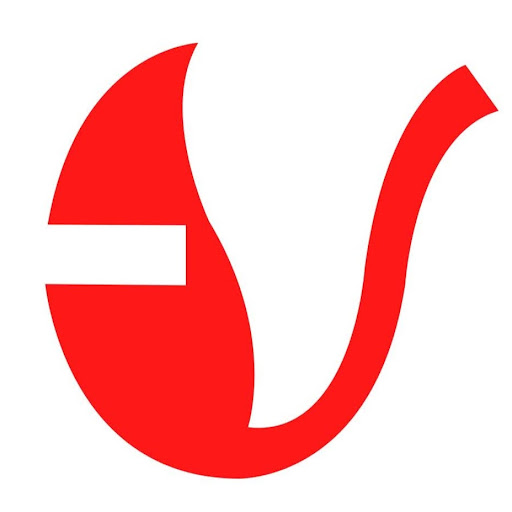# What will come at the place of question mark ?8, 28, 116, 584, ?

A. 1752

B. 3504

C. 3508

D. 3502

### Solution(By Examveda Team)

(8 *3) + 4 = 28 (28 *4) + 4 = 116 (116 *5) + 4 = 584 (584 *6) + 4 = 3508.

1.(116*4)+4=116 how is it

2.It was a mistake. Now Corrected. Thanks to spot it.

3.In question it 116,but in explanation 166????

4.c

5.7, 6, 10, 21.5, 53, ___